# Texas Go Math Grade 1 Lesson 13.2 Answer Key Identify Related Facts

Refer to our Texas Go Math Grade 1 Answer Key Pdf to score good marks in the exams. Test yourself by practicing the problems from Texas Go Math Grade 1 Lesson 13.2 Answer Key Identify Related Facts.

## Texas Go Math Grade 1 Lesson 13.2 Answer Key Identify Related Facts

Explore

Useto show 4 + 9 = 13.
Drawto show a related subtraction fact.
Write the subtraction sentence.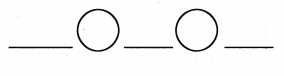13 – 9 = 4

FOR THE TEACHER • Read the following problem. Brandon walks 9 miles in the morning. He walks 4 miles in the evening. How many miles does Brandon walk in a day? Write the related facts.
Given that
Brandon walks in the morning = 9 miles
Brandon walks in the evening = 4 miles
Brandon walk in a day = 9 + 4 = 13
Math Talk
Mathematical Processes

Explain why your subtraction sentence is related to 4 + 9 = 13.
13 – 9 = 4 is related to the 4 + 9 = 13 because in 13 – 9 = 4 the number 9 send to the RHS side then we get 13 = 4 + 9.

Model and Draw

Use the pictures. What two facts can you write?These are related facts. If you know one of these facts, you also know the other fact.
3 + 9 = 12
12 – 3 = 9.

Share and Show

Add and subtract. Circle the related facts.

Question 1.
6 + 4 = _________
10 – 4 =________
6 + 4 = 10
10 – 4 = 6

Question 2.
_______ = 9 + 8
________ = 17 – 8
17 = 9 + 8
9 = 17 – 8

Question 3.
9 + 5 = __________
9 – 5 = _________
9 + 5 = 14
9 – 5 = 4

Question 4.
8 + 7 = ___________
15 – 7 = ___________
8 + 7 = 15
15 – 7 = 8

Question 5.
_________ = 9 + 2
_________ = 9 – 2
11 = 9 + 2
7 = 9 – 2

Question 6.
6 + 3 = __________
12 – 3 = ___________
6 + 3 = 9
12 – 3 = 9

Question 7.
4 + 8 = ___________
12 – 8 = ___________
4 + 8 = 12
12 – 8 = 4

Question 8.
_________ = 7 + 6
________ = 13 – 6
13 = 7 + 6
7 = 13 – 6

Question 9.
9 + 9 = ___________
18 – 9 = _________
9 + 9 = 18
18 – 9 = 9

Problem Solving

Question 10.
Use these numbers to write the related facts. 8 9 178 + 9 = 17
17 – 9 = 8
17 – 8 = 9

Question 11.
H.O.T. Which number can not be used to write related number sentences? Explain.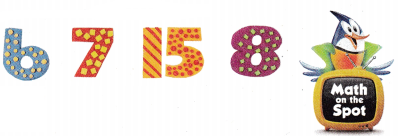9 is used to wi in the related number sentences.

Explanation
15 – 8 = 7
15 – 7 = 8
15 – 6 = 9
Therefore
15 – 9 = 6
So 9 is the related number to the number of sentences

H.O.T. Multi-Step Use the numbers to write related addition and subtraction sentences.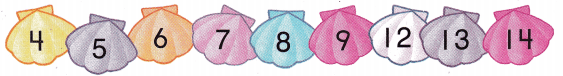Question 12.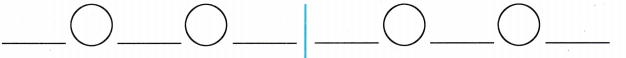9 + 5 = 14
12 – 4 = 8

Question 13.7 + 6 = 13
13 – 6 = 7

Question 14.
Analyze 11 elephants are washing themselves. Some elephants stop. 5 elephants keep washing themselves. Which fact is not related?
(A) 11 – 6 = 5
(B) 5 + 6 = 11
(C) 6 – 5 = 1
Given that
Elephants washing themselves = 11
Elephant keep washing themselves = 5
Some of the elephants are stop = 11 – 5 = 6
Total number of elephants = 11 + 5 = 16
6 – 5 is not related to the fact.
Option C is the correct answer.

Question 15.
9 elephants have their trunks up. 7 elephants do not have their trunks up. Which fact does not belong?(A) 9 + 7 = 16
(B) 16 – 6 = 10
(C) 16 – 9 = 7

Given that
Elephant have their trunks up = 9
Elephant do not have trunks up = 7
Total number of elephants = 16
16 – 6 is not related to the fact.

Question 16.
Jill has a garden. There are 4 roses. There are also 8 tulips. Which fact is not related?(A) 8 – 4 = 4
(B) 4 + 8 = 12
(C) 12 – 8 = 4
Given that
Total number of roses in the Jill garden = 4
Total number of tulips in the Jill garden = 8
Total number of flowers = 8 + 4 = 12
8 – 4 = 4 is not related to the fact.

Question 17.
Texas Test Prep Which subtraction fact is related to 6 + 8 = 14?
(A) 14 – 7 = 7
(B) 14 – 8 = 6
(C) 14 – 9 = 5
6 + 8 = 14 is related to 14 – 8 = 6
Option B is the correct answer

TAKE HOME ACTIVITY • Write 7, 9, 16, +, -, and = on separate slips of paper. Have your child use the slips of paper to show related facts.

### Texas Go Math Grade 1 Lesson 13.2 Homework and Practice Answer Key

Add and subtract. Circle the related facts.

Question 1.
8 + 5 = _________
13 – 5 = __________
8 + 5 = 13
13 – 5 = 8

Question 2.
4 + 3 = __________
10 – 3 = __________
4 + 3 = 7
10 – 3 = 7

Question 3.
9 + 4 = ___________
9 – 4 = ___________
9 + 4 = 13
9 – 4 = 5

Problem Solving

Question 4.
Which number can not be used to write related number sentences? Explain.
5 7 9 14
9 + 5 = 141
4 – 5 = 9
Number 7 is not related to the number sentences.
Explanation
In the number sentences adding or subtracting of numbers are related to 5, 9, 14, and number 7 is not related.

Multi-Step Use the numbers to write related addition and subtraction sentences.Question 5.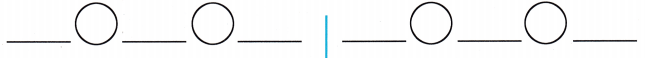4 + 5 = 9
8 + 5 = 13

Question 6.6 + 9 = 15
7 + 4 = 11

Lesson Check

Question 7.
13 squirrels are looking for nuts. Some squirrels stop. 6 squirrels keeping looking for nuts. Which fact is not related?(A) 13 – 7 = 6
(B) 7 – 6 = 1
(C) 6 + 7 = 13
Given that
Total number of squirrels looking for nuts = 13
Some of the squirrels are kept looking for nuts = 6
Total number of squirrels are stopped = 13 – 6 = 7
7 – 6 = 1 is not related
Option B is correct.

Question 8.
8 cats are sleeping. 9 cats are not sleeping. Which fact does not belong?(A) 9 + 8 = 17
(B) 17 – 9 = 8
(C) 17 – 7 = 10
Given that
Total number of cats sleeping = 8
Total number of cats not sleeping = 9
Total number of cats = 8 + 9 = 17
17 – 7 = 10 is not belonging to the fact.
Option C is correct.

Question 9.
Which subtraction fact is related to 8 + 3 = 11?
(A) 8 – 3 = 5
(B) 11 – 7 = 2
(C) 11 – 8 = 3
8 + 3 = 11 is related to the subtraction 11 – 8 = 3.

Option C is the correct answer.

Question 10.
Multi-step Mike has 5 soccer balls and 7 baseballs. Which number can not be used to write related number sentences with 5 and 7?(A) 8
(B) 5
(C) 12